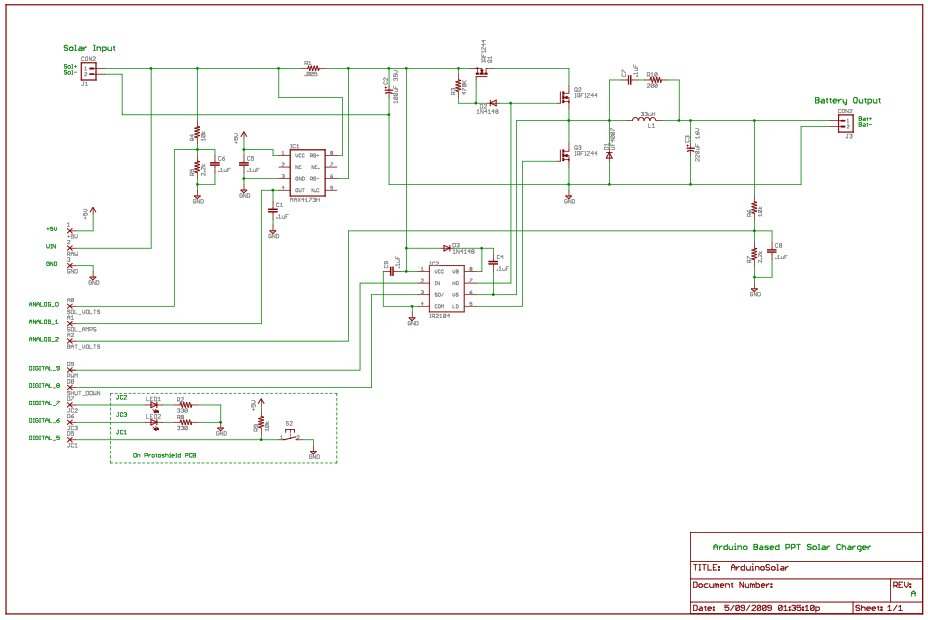# Arduino Peak Power Tracker Solar Charger

This project is an update of my original Peak Power Tracker Battery Charger Project. It is designed to control a 12V solar panel charging a 12V lead acid battery. The updated version of this project uses the Arduino Duemilanove development board (from www.sparkfun.com) as the basis for the project. Then I used the Arduino Protoshield Kit (also from www.sparkfun.com) to contruct the charging circuit and mate it to the processor board.PPT Charger Hardware Description: ArduinoSolarHardware.txt ArduinoSolarHardware.rtf

PPT Charger Parts List: ArduinoSolarPartsList.txt

PPT Charger Software(Arduino Sketch): ppt.pde

PPT Charger Photos: Duemilanove and Protoshield, Prototshield back, Protoshield front.

To explain how Peak Power Tracking works I’ve printed part of my original article that I wrote for Home Power Magazine. (Link to full article – Peak Power Tracking article.pdf)

To understand why the PPT can increase the efficiency of your solar power charging system a closer at the electrical characteristics of a solar panel is necessary. Solar panels convert photons from the sun striking their surfaces into electricity of a characteristic voltage and current. The solar panel’s electrical output can be plotted on a graph of voltage vs. current: an IV curve. I represents the current in amps and V represents the voltage in volts. The resulting line on the graph shows the current output of the panel for each voltage at a specific light level and temperature. (Fig. 2) The current is constant until reaching the higher voltages, when it falls off rapidly. This IV curve is applicable to the electrical output of all solar panels.

READ  Xbox 360 RF module + Arduino

However, in a solar power system we are more concerned with the power we can get out of the system, power we can use to do useful work. In an electrical system power is measured in watts, which is the product of the voltage and current (W = I x V) generated by the panel. Graphing the watts generated by the solar panel shows an interesting characteristic: the maximum watts are produced at a panel voltage of about 18v. This value is called the Maximum Power Point or MPP. Since the goal of the PPT to generate the maximum power from the solar panels, operating the solar panels at roughly this voltage is optimal. However, when a solar panel is used to charge a 12v battery directly, the battery pulls the operating voltage of the panel down to its own voltage of 12v. As shown on the graph, the solar panel is producing significantly less power (watts) at 12v than at 18v. So here is an opportunity to gain more power out of the solar panel charging system if the solar panel continues to operate at 18v while charging a 12v battery.

To gain the efficiency of Peak Power Tracking, the 18v of the solar panel must be converted to the 12v of the battery. This can be accomplished by using an electronic circuit called a DC/DC converter. A DC/DC converter is a very common device found in most DC power supplies in some form. It is the basis of the PPT. The DC/DC converter changes the solar panel’s higher voltage and lower current to the lower voltage and higher current needed to charge the battery. Because the DC/DC converter is theoretically a loss-less device (less some small real world inefficiencies), it outputs the same amount of watts as are input, but at a different voltage and current. In a power supply, simple feedback is used to set the DC/DC converter to a fixed output voltage. This is done by controlling the ratio of the input voltage to the output voltage. In the solar panel example, the ratio would be 18v/12v or 3/2.

READ  Fun Shway Display using an ArduinoHowever, for any solar panel, the Maximum Power Point is not fixed. Consider the IV curves for any solar panel; (E0004X.pdf) the graph will show that the curves change with the amount of light and the temperature of the panel. They also change for each individual solar panel. As the curves change, the MPP changes for the different temperatures and light levels. If the MPP changes, the conversion ratio of the input voltage to output voltage of DC/DC converter must also change to keep the solar panel voltage at the MPP.

### Major Components in Project

Arduino Duemilanove Development Board DEV-00666

Arduino ProtoShield Kit DEV-07914

For more detail: Arduino Peak Power Tracker Solar Charger

#### This Post / Project can also be found using search terms:

• arduino solar charger
• sun tracking solar panel arduino code
• arduino peak power tracker
• arduino solar li ion charger